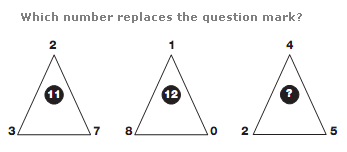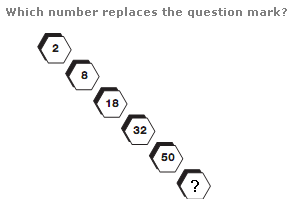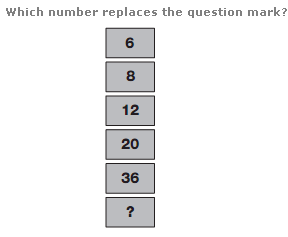# Puzzles - Number puzzles

Exercise : Number puzzles
85.:
9
Explanation
:
Working clockwise around the 3 triangles, the sum of the outer digits in each triangle is written in the centre of the triangle one place clockwise.

86.:
72
Explanation
:
Numbers follow the sequence of Square Numbers, from 1 to 6, multiplying each one by 2.

87.